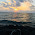## Search This Blog

THE BEAUTY OF MATHEMATICS- Collected from The Internet and Various Books to enrich The students and Teachers. SUPPORT with YOUR COMMENTS ...

## Monday, October 19, 2009

### JOHANN CARL FRIEDRICH GAUSS

Johann Carl Friedrich Gauss , a German mathematician who had a remarkable influence in many fields , including number theory, statistics, analysis, differential geometry, electrostatics, astronomy and optics is ranked as one of history's most influential mathematicians.
CHILD PRODIGY:

At the age of three he amazed his father by correcting an arithmetical error.

In primary school his teacher, J.G. Büttner, tried to occupy pupils by making them add a list of integers. The young Gauss reputedly produced the correct answer within seconds, to the astonishment of his teacher.

Gauss's presumed method, which supposes the list of numbers was from 1 to 100, was to realize that pairwise addition of terms from opposite ends of the list yielded identical intermediate sums:

1 + 100 = 101, 2 + 99 = 101, 3 + 98 = 101, and so on, for a total sum of 50 × 101 = 5050 .

FAMOUS QUOTE:
“Mathematics is the queen of the sciences and number theory is the queen of mathematics”
“Ask her to wait a moment,I am almost done” (he told this while working when he was informed that his wife is dying).
CONTRIBUTIONS:

• In Disquisitiones Arithmeticae, one of the most brilliant achievements in mathematics, Gauss systematized the study of number theory. This work was fundamental in consolidating number theory as a discipline and has shaped the field to the present day.
• Gauss proved the Fundamental Theorem of Algebra, which states that every polynomial has a root of the form a+bi.
• He also discovered the Cauchy Integral theorem for analytic functions
• Gauss's work in mathematical physics contributed to potential theory and the development of the Principle of Conservation of Energy.
• Theoria motus corporum celestium (theory of motion of the celestial bodies) is his most significant work on applied mathematics.
• Gauss discovered Ceres, the largest of the asteroids orbiting around the Sun.
• His Theory of Celestial Movement remains a cornerstone of astronomical computation. It introduced the Gaussian gravitational constant.
• Introduced the Method of Least Squares, a procedure used in all sciences to this day to minimize the impact of measurement error.

1.thanks for the info...

1.2.he also coined the term "heureka!"

3.thisss info wass rewlly qwwwwd usee

4.Anne is WRONG! ARCHIMEDES coined the term 'eureka.' and anne spelled it wrong!

5.anonymous is right, it was archimedes who coined the term eureka which mean "i have found it !"

6.yes it is Archimedes who coined the term eureka which mean "i have found it !"

http://malini-math.blogspot.com/2009/11/archimedes-father-of-mathematics.html

7.Thank you I really needed this info

8.you helped me a lot in my project.

9.thank you for helping me also

10.11.THANKZ A LOT ,for the help

12.Thanx for d related information which helped me a lot

13.THz this info was rlly useful!! :) ;) :{)

14.that was some good info

15.nice info rally helped me

16.17.It was very useful for me...thanks

18.This comment has been removed by a blog administrator.

19.